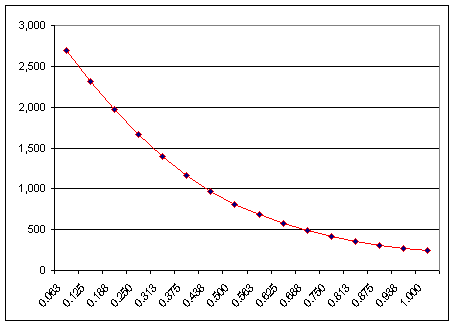# How does distance affect magnetic force?

Aug 22, 2014

Magnetic force obeys an inverse square law with distance.

The equation for magnetic force is similar to Coulomb's Law (if you are familiar with it). But the key point is that the force is inversely proportional to the distance squared (i.e. it obeys an inverse square law with distance).
$\left(F \propto \frac{1}{r} ^ 2\right)$
Where $r$ is the distance between the magnets.

What that means in practical terms is the following:

1. If the distance between two magnets is doubled the magnetic force between them will fall to a quarter of the initial value.
$\left(F / 4\right)$
2. If the distance between two magnets is halved the magnetic force between them will increase to four times the initial value.
$\left(4 F\right)$
3. If the distance between two magnets is increased by five times the magnetic force between them will fall to one twenty fifth of the initial value.
$\left(F / 25\right)$